Theoretical study of a mechanical device with a non-zero momentum | Статья в журнале «Молодой ученый»

Отправьте статью сегодня! Журнал выйдет 7 октября, печатный экземпляр отправим 11 октября.

Опубликовать статью в журнале
Проекты
Меню

Рубрика: Физика

Дата публикации: 06.12.2020

Статья просмотрена: 161 раз

### Библиографическое описание:

Филенко, А. В. Theoretical study of a mechanical device with a non-zero momentum / А. В. Филенко. — Текст : непосредственный // Молодой ученый. — 2020. — № 49 (339). — С. 3-7. — URL: https://moluch.ru/archive/339/76149/ (дата обращения: 23.09.2023).

﻿

This article presents a theoretical study of a mechanical device that creates a momentum without the exhaust of a propellant.

Key words: impulse, centripetal force, propellantless drive, reactionless drive.

Introduction

To study the operation of the device and the momentum created by it, from the point of view of the author of the article, it is advisable to use a physical quantity which is the impulse . This value, which is a change in the momentum, can show the operability and efficiency of the device considered below.

To understand the essence of the processes considered, calculations are given under ideal conditions, which are:

— friction force is not taken into account,

— the effect of the force of gravity is not taken into account,

— transients (symmetrical or insignificant) are not taken into account.

However, generally accepted physical concepts, forces and formulas are used.

Consider a device with rotating satellites around a central body and changing the radius of motion from R 1 to R 2 and back. Consider the trajectory of the satellites shown in the diagram (see Figure 1). The main condition for the operation of the device is to maintain a constant angular velocity (ω-const).

Let's denote the mass of the central body as m1, the mass of the satellite m 2 . In the calculations, signs are immediately marked in accordance with the direction of the X and Y axes. The connection between the central body and the satellite will be considered rigid. All further calculations were made relative to the central body. The calculations are made for the upper half of the device and only the projection on the X-axis is taken into account. The projection on the Y-axis is not taken into account due to the symmetrical and oppositely directed operation of the upper and lower half of the device.

Let us investigate the change in the momentum at different time intervals of the presented device. When the satellite moves along the trajectory 1–2, the Coriolis force will act on the device. The Coriolis force is calculated by the formula (1),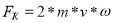(1)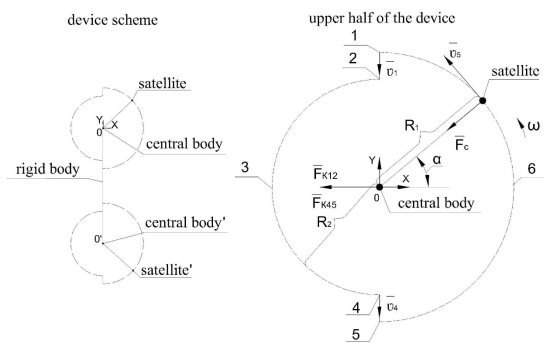Fig. 1

The vector F K12 is transferred and the direction of impact on the central body is indicated (see Figure 1), as a result, the change in momentum on trajectory 1–2 can be found by the following formula (2).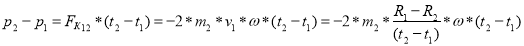(2)

The transformation result is as follows (3).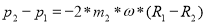(3)

Let us denote the period of the complete revolution of the satellite around the central body (4),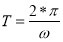(4)

Next, let's consider the movement of the satellite along an arc 2–3–4 with a radius R 2 and the projection of the change in momentum on the X-axis is expressed as follows (5),(5)

The transformation result is as follows (6).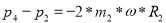(6)

In the interval 4–5, the change in the momentum will be according to the same formula as in the interval 1–2 (see the formula (6). Most importantly, the direction of the change in the momentum will be directed in the same direction for the change in the momentum 1–2 and the change in the momentum 4 -5 (see ther formula (7).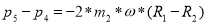(7)

Next, let's consider the movement of the satellite along an arc 5–6–7 with a radius R1 and express the projection of the change in momentum on the X-axis, for this we will use formulas (5) and (6), replace R 2 with R 1 and obtain (8).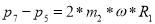. (8)

Now, knowing the momentum change at each separate section of the satellite's trajectory, we can find the momentum change over the entire cycle of the device, i.e. for one turnover of the satellite (9).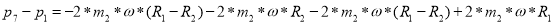(9)

The total change in momentum for one cycle (revolution) of the satellite will be expressed as follows (10).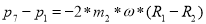(10)

The minus sign in expression (10) indicates that the change in momentum is directed to the left (see Figure 1), which corresponds to the direction in the direction of the arc trajectory with a smaller radius.

It should be borne in mind that the diagram of the device (see Figure 1) is an ideal trajectory that is not achievable in real conditions. For example, if the angular velocity is constant (ω-const), movement 1–2 along the specified direction is impossible, since this movement takes time. Accordingly, it should be understood that expression (10) is not achievable, but shows the theoretical maximum of the pulse change that can be achieved from the considered device scheme.

Calculation of K. E. Tsiolkovsky, the scientist of reactionless drive .

Let us suppose that the device is located that way that gravity does not create any forces in the device itself.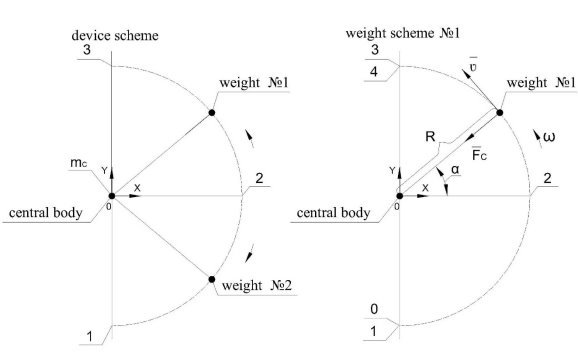Fig. 2

Let's consider the work of the device weights separately, take the weight 1, see Figure 2. Let's denote m с which is the mass of the central body, m 1 is the mass of the weight 1, m 2 is the mass of the weight 2 and m 1 = m 2 = m. Let's set that at points 0 and 4 the momentum of the weight will be equal to zero, p 0 = 0 and p 4 = 0. At point 0, weight 1 is given a momentum p 1 = m * ʋ, exactly the same magnitude but directed in the opposite direction, the momentum will receive the device itself (the central body). Further, when moving along the trajectory 1–2–3, weight 1 will make a change in momentum caused by the centripetal force F С , projected onto the X-axis, calculated as follows:(11)

And next we transform this: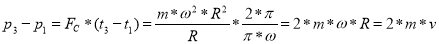(12)

Now at point 3, in order to stop weight. 1, it is necessary to apply an momentum p 3 = m * ʋ. Let’s calculate the total change in momentum in the segment 1–3, then we put the signs in relation to the central body of the device: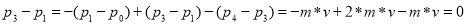(13)

The resulting change in momentum over a full cycle is zero, and no movement due to symmetrical movement occurs.

Conclusions.

The above calculation, for the diagram of a mechanical device (see Figure 1), was made using generally accepted physical laws and quantities. The calculation result shows that the pulse of the device for a complete cycle is not equal to zero. The above calculation shows the possibility of creating reactionless drive operating without the exhaust of a propellant.

References:

1. Impulse — Wikipedia. [Electronic resource] Access mode: ru.wikipedia.org ›wiki (Date of treatment 09/28/2020)
2. Okolotin V. In search of reactionless drive // Science and technology [Electronic resource] Access mode: http://n-t.ru/tp/ts/pi.htm (Date of treatment 09/28/2020)

## Ключевые слова

impulse, centripetal force, propellantless drive, reactionless drive
Задать вопрос